# Introduction

Sensitivity (or recall or true positive rate), false positive rate, specificity, precision (or positive predictive value), negative predictive value, misclassification rate, accuracy, F-score- these are popular metrics for assessing performance of binary classifier for certain threshold. These metrics are calculated at certain threshold values. Receiver operating characteristic (ROC) curve is a common tool for assessing overall diagnostic ability of the binary classifier. Unlike depending on a certain threshold, area under ROC curve (also known as AUC), is a summary statistic about how well a binary classifier performs overall for the classification task. ROCit package provides flexibility to easily evaluate threshold-bound metrics. Also, ROC curve, along with AUC can be obtained using different methods, such as empirical, binormal and non-parametric. ROCit encompasses a wide variety of methods for constructing confidence interval of ROC curve and AUC. ROCit also features the option of constructing empirical gains table, which is a handy tool for direct marketing. The package offers options for commonly used visualization, such as, ROC curve, KS plot, lift plot. Along with in-built default graphics setting, there are rooms for manual tweak by providing the necessary values as function arguments. ROCit is a powerful tool offering a range of things, yet it is very easy to use.

# Binary Classifier

In statistics and machine learning arena, classification is a problem of labeling an observation from a finite number of possible classes. Binary classification is a special case of classification problem, where the number of possible labels is two. It is a task of labeling an observation from two possible labels. The dependent variable represents one of two conceptually opposed values (often coded with 0 and 1), for example:

• the outcome of an experiment- pass (1) or fail (0)
• the response of a question- yes (1) or no (0)
• presence of some feature- absent (0) or present (1)

There are many algorithms that can be used to predict binary response. Some of the widely used techniques are logistic regression, discriminant analysis, Naive Bayes classification, decision tree, random forest, neural network, support vector machines (James et al. 2013), etc. In general, the algorithms model the probability of one of the two events to occur, for the certain values of the covariates, which in mathematical terms can be expressed as $$Pr(Y=1|X_1=x_1, X_2=x_2,\dots,X_n=x_n)$$. Certain threshold can then be applied to convert the probabilities into classes.

# Binary Classifier Performance Metrics

## Hard Classification

When hard classification are made, (after converting the probabilities using threshold or returned by the algorithm), there can be four cases for a certain observation:

1. The response actually negative, the algorithm predicts it to be negative. This is known as true negative (TN).

2. The response actually negative, the algorithm predicts it to be positive. This is known as false positive (FP).

3. The response actually positive, the algorithm predicts it to be positive. This is known as true positive (TP).

4. The response actually positive, the algorithm predicts it to be negative. This is known as false negative (FN).

All the observations fall into one of the four categories stated above and form a confusion matrix.

Predicted Negative (0) Predicted Positive (1)
Actual Negative (0) True Negative (TN) False Positive (FP)
Actual Positive (1) False Negative (FN) True Positive (TP)

Following are some popular performance metrics, when observations are hard classified:

• Misclassification: Misclassification rate, or error rate is the most common metric used to quantify a binary classifier. This is the probability that the classifier makes a wrong prediction, which can be expressed as: $Misclassification\ rate=Pr(\hat{Y}\neq Y)=\frac{FN+FP}{TN+FN+TP+FP}$

• Accuracy: This simply accounts for the number of correct classifications made. $Accuracy = Pr(\hat{Y}=Y)=1-Misclassification\ rate$

• Sensitivity: Sensitivity measures the proportion of the positive responses that are correctly identified as positive by the classifier (Altman and Bland 1994a). In other words, it is the true positive rate and can be calculated directly from the entries of confusion matrix. $Sensitivity=Pr(\hat{Y}=1|Y=1)=\frac{TP}{TP+FN}$ Other terms used to represent the same metric are true positive rate (TPR), recall. The term sensitivity is popular in medical test (Altman and Bland 1994b). In the credit world the use of the term TPR has been noticed (Siddiqi 2012). While in machine learning, natural language processing, use of recall is common (Nguyen and Armitage 2006; Denecke 2008; Bermingham and Smeaton 2011, @huang2009analyzing)

• Specificity: Specificity measures the proportion of the negative responses that are correctly identified as negative by the classifier (Altman and Bland 1994a). In other words, it is the true negative rate and can be calculated directly from the entries of confusion matrix. $Specificity=Pr(\hat{Y}=0|Y=0)=\frac{TN}{TN+FP}$

Specificity is also known as true negative rate (TNR).

• Positive predictive value (PPV): Positive predictive value (PPV) is the probability that an observation classified as positive is truly positive. It can be calculated from the entries of confusion matrix: $PPV=Pr(Y=1|\hat{Y}=1)=\frac{TP}{TP+FP}$

• Negative predictive value (NPV): Negative predictive value (NPV) is the probability that an observation classified as negative is truly negative. It can be calculated from the entries of confusion matrix. $NPV=Pr(Y=0|\hat{Y}=0)=\frac{TN}{TN+FN}$

• Diagnostic likelihood ratio (DLR): Likelihood ratio is another form of accuracy measure of a binary classifier. This is a ratio. From true statistical sense, this ratio is a likelihood ratio, but in the context of accuracy measure, this is called diagnostic likelihood ratio (DLR) (Pepe 2003). There are two kinds of DLR metrics are defined:

$Positive\ DLR=\frac{TPR}{FPR}$ $Negative\ DLR=\frac{TNR}{FNR}$

• F-Score: F-Score (also known as F-measure, F1 -Score) is another metric which is used to assess the performance of a binary classifier. It is often used in information theory, to assess search, document classification, and query classification performance (Beitzel et al. 2007). It is defines as the harmonic mean of precision (Positive predictive value, PPV) and recall (True positive rate, TPR).

$F\text{-}Score=\frac{2}{\frac{1}{PPV} +\frac{1}{TPR}}=2\times \frac{PPV\times TPR}{PPV+TPR}$

## Observation Are Scored

Rather than making simple classification, often models give probability scores, $$Pr(Y=1)$$. Using certain cutoff or threshold values, we can dichotomize the scores and calculate these metrics. This is also true when some certain diagnostic variable is used to categorize the observations. For example, having a hemoglobin A1c level of lower than 6.5% being treated as no diabetes, and having a level equal to greater than 6.5% being treated as having the disease. Here the diagnostic measure is not bound in between 0 and 1 like the probability measure, yet all the metrics stated above can be derived. But these metrics give a sense of performance measure only at certain threshold. There are metrics, that measure overall performance of the binary classifier considering the performance at all possible thresholds. Two such metrics are

1. Area under receiver operating characteristic (ROC) curve
2. KS statistic

Receiver operating characteristic (ROC) curve (Lusted 1971, @hanley1982meaning, @bewick2004statistics) is a simple yet powerful tool used to evaluate a binary classifier quantitatively. The most common quantitative measure is the area under the curve (Hanley and McNeil 1982). ROC curve is drawn by plotting the sensitivity (TPR) along $$Y$$ axis and corresponding 1-specificity (FPR) along $$X$$ axis for all possible cutoff values. Mathematically, it is the set of all ordered pairs $$(FPR(c), TPR(c))$$, where $$c\in R$$.

Some Properties of ROC curve

• ROC curve is a monotonically increasing function, defined in the $$(+,+)$$ quadrant.

• ROC curve is that it is invariant of strictly increasing transformation of the diagnostic variable, when estimated empirically.

• The ROC curve always contains $$(0,0)$$ and $$(1,1)$$. These are the extreme points when the threshold is set to $$+\infty$$ and $$-\infty$$.

If the diagnostic variable is unrelated with the binary outcome, the expected ROC curve is simply the $$y=x$$ line. In a situation where the diagnostic variable can perfectly separate the two classes, the ROC curve consists of a vertical line ($$x=0$$) and a horizontal line ($$y=1$$). For a practical data, usually the ROC stays in between these two extreme scenarios. Figure below illustrates some examples of different types of ROC curves. The red and the green curves illustrate two extreme scenarios. The random line in red is the expected ROC curve when the diagnostic variable does not have any predictive power. When the observations are perfectly separable, the ROC curve consists of one horizontal and a vertical line as shown in green. The other curves are the result of typical practical data. When the curve shifts more to the north-west, it means better the predictive power.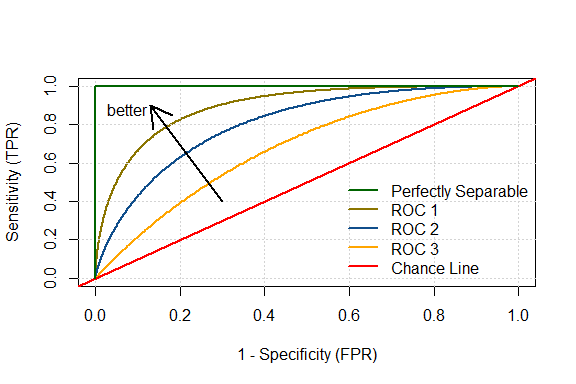ROC curves example

For more details, see Pepe (2003).

### Common approaches to estimate ROC curve

• Empirical: The empirical method simply constructs the ROC curve empirically, applying the definitions of TPR and FPR to the observed data. Figure 1 is an example of such approach. For every possible cutoff value c, TPR and FPR are estimated by:

$\hat{TPR}(c)=\sum_{i=1}^{n_Y}I(D_{Y_i}\geq c)/n_Y$

$\hat{FPR}(c)=\sum_{j=1}^{n_{\bar{Y}}}I(D_{{\bar{Y}}_j}\geq c)/n_{\bar{Y}}$ where, $$Y$$ and $$\bar{Y}$$ represent the positive and negative responses, $$n_Y$$ and $$n_{\bar{Y}}$$ are the total number of positive and negative responses, $$D_Y$$ and $$D_{\bar{Y}}$$ are the distributions of the diagnostic variable in the positive and the negative responses. The indicator function has the usual meaning. It evaluates 1 if the expression is true, and 0 otherwise. The area under empirically estimated ROC curve is given by:

$\hat{AUC}=\frac{1}{n_Yn_{\bar{Y}}} \sum_{i=1}^{n_Y}\sum_{j=1}^{n_{\bar{Y}}} (I(D_{Y_i}>D_{Y_j})+ \frac{1}{2}I(D_{Y_i}>D_{Y_j}))$ The variance of AUC can be estimated as (Hanley and McNeil 1982): $V(AUC)=\frac{1}{n_Yn_{\bar{Y}}}( AUC(1-AUC) + (n_Y-1)(Q_1-AUC^2) + (n_{\bar{Y}}-1)(Q_2-AUC^2) )$ where, $$Q_1=\frac{AUC}{2-AUC}$$, and $$Q_2=\frac{2\times AUC^2}{1+AUC}$$.

An alternate formula is developed by DeLong, DeLong, and Clarke-Pearson (1988) which is given in terms of survivor functions: $V(AUC)=\frac{V(S_{D_{\bar{Y}}}(D_Y))}{n_Y} +\frac{V(S_{D_Y}(D_{\bar{Y}}))}{n_{\bar{Y}}}$

A confidence band can be computed using the usual approach of normal assumption. For example, a $$(1-\alpha)\times 100\%$$ confidence band can be constructed using:

$AUC\pm\phi^{-1}(1-\alpha/2)\sqrt{V(AUC)}$

The above formula does not put any restriction on the computed values of upper and lower bound. However, AUC is a measure bounded between 0 and 1. One systematic way to do this is the logit transformation (Pepe 2003). Instead of constructing the interval directly for the AUC, an interval in the logit scale is first constructed using:

$L_{AUC}\pm \phi^{-1}(1-\alpha/2)\frac{\sqrt{AUC}}{AUC(1-AUC)}$

where $$L_{AUC}=log(\frac{AUC}{1-AUC})$$ is the logit of AUC. The logit scale intervals can then be inverse logit transformed to find the actual bounds of AUC.

Confidence interval of ROC curve: For large values of $$n_Y$$ and $$n_{\bar{Y}}$$, the distribution of $$TPR(c)$$ at $$FPR(c)$$ can be approximated as a normal distribution with following mean and variance:

$\mu_{TPR(c)}=\sum_{i=1}^{n_Y}I(D_{Y_i}\geq c)/n_Y$

$V \Big( TPR(c) \Big)= \frac{ TPR(c) \Big( 1- TPR(c)\Big) }{n_Y} + \bigg( \frac{g(c^*)}{f(c^*) } \bigg)^2\times K$ where, $K=\frac{ FPR(c) \Big(1-FPR(c)\Big)}{n_{\bar{Y}} }$

$c^*=S^{-1}_{D_{\bar{ Y}}}\Big( FPR(c) \Big)$ and, $$S$$ is the survival function given by, $S(t)=P\Big(T>t\Big)=\int_t^{\infty}f_T(t)dt=1-F(t)$ For details, see Pepe (2003).

• Binormal: This is a parametric approach where the diagnostic variable in the two groups are assumed to be normal.

$D_Y\sim N(\mu_{D_Y}, \sigma_{D_Y}^2)$

$D_{\bar{Y}}\sim N(\mu_{D_{\bar{Y}}}, \sigma_{D_{\bar{Y}}}^2)$

When such distributional assumptions are made, ROC curve can be defined as:

$y(x)=1-G(F^{-1}(1-x)), \ \ 0\leq x\leq 1$ where by $$F$$ and $$G$$ are the cumulative density functions of the diagnostic score in the negative and positive groups respectively, with $$f$$ and $$g$$ being corresponding probability density functions. For normal condition, the ROC curve and AUC under curve are given by:

$ROC\ Curve: y= \phi(A+BZ_x)$

$AUC=\phi(\frac{A}{\sqrt{1+B^2}})$

where, $$Z_x=\phi^{-1}(x(t))=\frac{\mu_{D_{\bar{Y}}}-t}{\sigma_{D_{\bar{Y}}}}$$, $$t$$ being a cutoff; and $$A=\frac{|\mu_{D_{{Y}}}-\mu_{D_{\bar{Y}}}|}{\sigma_{D_{{Y}}}}$$, $$B=\frac{\sigma_{D_{\bar{Y}}}}{\sigma_{D_{{Y}}}}$$.

Confidence interval of ROC curve: To get the confidence interval, variance of $$A+BZ_x$$ is derived using:

$V(A+B Z_x)=V(A)+Z_x^2V(B)+2Z_xCov(A, B)$ A $$(1-\alpha)\times100\%$$ level confidence limit for $$A+Z_xB$$ can be obtained as

$(A+Z_xB)\pm \phi^{-1}(1-\alpha/2)\sqrt{V(A+Z_xB)}$ Point-wise confidence limit can be achieved by taking $$\phi$$ of the above expression.

• Non-parametric: Non-parametric estimates of $$f$$ and $$g$$ are used in this approach. Zou, Hall, and Shapiro (1997) presented one such approach using Kernel densities:

$\hat{f}(x)=\frac{1}{n_{\bar{Y}}h_{\bar{ Y}}}\sum_{i=1}^{n_{\bar{ Y}}} K\big( \frac{x-D_{\bar{ Y}i} }{h_{\bar{ Y}}} \big)$

$\hat{g}(x)= \frac{1}{n_{{Y}}h_y}\sum_{i=1}^{n_{{ Y}}} K\big( \frac{x-D_{{ Y}i} }{h_Y} \big)$

where $$K$$ is the Kernel function and $$h$$ smoothing parameter (bandwidth). Zou, Hall, and Shapiro (1997) suggested a biweight Kernel:

$K\big(\frac{x-\alpha}{\beta}\big)=\begin{cases} \frac{15}{16} \Big[ 1-\big(\frac{x-\alpha}{\beta}\big)^2 \Big] , & x\in (\alpha - \beta, \alpha + \beta)\\ 0, & \text{otherwise} \end{cases}$

with the bandwidth given by, $h_{\bar{Y}}=0.9\times min\big( \sigma_{\bar{ Y}}, \frac{IQR(D_{\bar{ Y}})}{1.34} \big)/ (n_{\bar{ Y}} )^{\frac{1}{5}}$ $h_{{Y}}=0.9\times min\big( \sigma_{{ Y}}, \frac{IQR(D_{{ Y}})}{1.34} \big)/ (n_{{ Y}} )^{\frac{1}{5}}$

Smoother versions of TPR and FPR are obtained as the right-hand side area (of cutoff) of the smoothed $$f$$ and $$g$$. That is,

$\hat{TPR}(t)=1-\int_{-\infty}^{t}\hat{g}(t)dt=1-\hat{G}(t)$

$\hat{FPR}(t)=1-\int_{-\infty}^{t}\hat{f}(t)dt=1-\hat{F}(t)$ When discrete pairs of $$(FPR, TPR)$$ are obtained, trapezoidal rule can be applied to calculate the AUC.

# Using Package ROCit

## 1/0 coding of response

A binary response can exist as factor, character, or numerics other than 1 and 0. Often it is desired to have the response coded with just 1/0. This makes many calculations easier.

library(ROCit)
data("Loan")

# check the class variable
summary(Loan$Status) #> CO FP #> 131 769 class(Loan$Status)
#>  "factor"

So the response is a factor variable. There are 131 cases of charged off and 769 cases of fully paid. Often the probability of defaulting is modeled in loan data, making the fully paid group as reference.

Simple_Y <- convertclass(x = Loan$Status, reference = "FP") # charged off rate mean(Simple_Y) #>  0.1455556 If reference not specified, alphabetically, charged off group is set as reference. mean(convertclass(x = Loan$Status))
#>  0.8544444

## Performance metrics of binary classifier

Various performance metrics for binary classifier are available that are cutoff specific. Following metrics can be called for via measure argument:

• ACC: Overall accuracy of classification.
• MIS: Misclassification rate.
• SENS: Sensitivity.
• SPEC: Specificity.
• PREC: Precision.
• REC: Recall. Same as sensitivity.
• PPV: Positive predictive value.
• NPV: Positive predictive value.
• TPR: True positive rate.
• FPR: False positive rate.
• TNR: True negative rate.
• FNR: False negative rate.
• pDLR: Positive diagnostic likelihood ratio.
• nDLR: Negative diagnostic likelihood ratio.
• FSCR: F-score,.
data("Diabetes")
logistic.model <- glm(as.factor(dtest)~chol+age+bmi,
data = Diabetes,family = "binomial")
class <- logistic.model$y score <- logistic.model$fitted.values
# -------------------------------------------------------------
measure <- measureit(score = score, class = class,
measure = c("ACC", "SENS", "FSCR"))
names(measure)
#>  "Cutoff" "Depth"  "TP"     "FP"     "TN"     "FN"     "ACC"    "SENS"
#>  "FSCR"
plot(measure$ACC~measure$Cutoff, type = "l")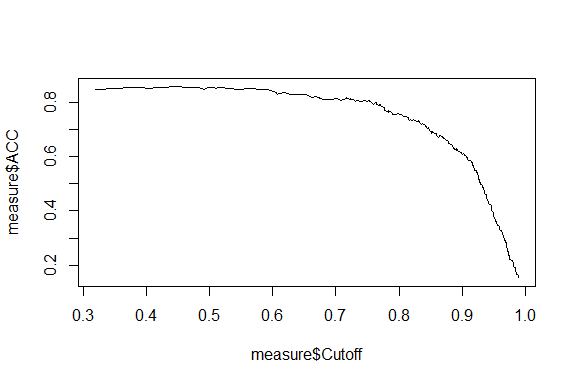Accuracy vs Cutoff

## ROC curve estimation

rocit is the main function of ROCit package. With the diagnostic score and the class of each observation, it calculates true positive rate (sensitivity) and false positive rate (1-Specificity) at convenient cutoff values to construct ROC curve. The function returns “rocit” object, which can be passed as arguments for other S3 methods.

Diabetes data contains information on 403 subjects from 1046 subjects who were interviewed in a study to understand the prevalence of obesity, diabetes, and other cardiovascular risk factors in central Virginia for African Americans. According to Dr John Hong, Diabetes Mellitus Type II (adult onset diabetes) is associated most strongly with obesity. The waist/hip ratio may be a predictor in diabetes and heart disease. DM II is also associated with hypertension - they may both be part of “Syndrome X”. The 403 subjects were the ones who were actually screened for diabetes. Glycosolated hemoglobin > 7.0 is usually taken as a positive diagnosis of diabetes.

In the data, the dtest variable indicates whether glyhb is greater than 7 or not.

data("Diabetes")
summary(Diabetes$dtest) #> Length Class Mode #> 403 character character summary(as.factor(Diabetes$dtest))
#>    +    - NA's
#>   60  330   13

The variable is a character variable in the dataset. There are 60 positive and 330 negative instances. There are also 13 instances of NAs.

Now let us use the total cholesterol as a diagnostic measure of having the disease.

roc_empirical <- rocit(score = Diabetes$chol, class = Diabetes$dtest,
negref = "-")
#> removing NA(s) from score and/or class
#> NA(s) removed

The negative was taken as the reference group in rocit function. No method was specified, by default empirical was used.

class(roc_empirical)
#>  "rocit"
methods(class="rocit")
#>  ciAUC      ciROC      gainstable ksplot     measureit  plot
#>  summary
#> see '?methods' for accessing help and source code

The summary method is available for a rocit object.

summary(roc_empirical)
#>
#>  Empirical ROC curve
#>  Number of postive responses :  60
#>  Number of negative responses :  329
#>  Area under curve :  0.652684903748734
# function returns
names(roc_empirical)
#>  "method"    "pos_count" "neg_count" "pos_D"     "neg_D"     "AUC"
#>  "Cutoff"    "TPR"       "FPR"
# -------
message("Number of positive responses used: ", roc_empirical$pos_count) #> Number of positive responses used: 60 message("Number of negative responses used: ", roc_empirical$neg_count)
#> Number of negative responses used: 329

The Cutoffs are in descending order. TPR and FPR are in ascending order. The first cutoff is set to $$+\infty$$ and the last cutoff is equal to the lowest score in the data that are used for ROC curve estimation. A score greater or equal to the cutoff is treated as positive.

head(cbind(Cutoff=roc_empirical$Cutoff, TPR=roc_empirical$TPR,
FPR=roc_empirical$FPR)) #> Cutoff TPR FPR #> [1,] Inf 0.00000000 0.000000000 #> [2,] 443 0.01666667 0.000000000 #> [3,] 404 0.03333333 0.000000000 #> [4,] 347 0.03333333 0.003039514 #> [5,] 342 0.05000000 0.003039514 #> [6,] 337 0.05000000 0.006079027 tail(cbind(Cutoff=roc_empirical$Cutoff,
TPR=roc_empirical$TPR, FPR=roc_empirical$FPR))
#>        Cutoff       TPR       FPR
#> [385,]    129 0.9666667 0.9908815
#> [386,]    128 0.9833333 0.9908815
#> [387,]    122 0.9833333 0.9939210
#> [388,]    118 0.9833333 0.9969605
#> [389,]    115 1.0000000 0.9969605
#> [390,]     78 1.0000000 1.0000000

Other methods:

roc_binormal <- rocit(score = Diabetes$chol, class = Diabetes$dtest,
negref = "-",
method = "bin")
#> removing NA(s) from score and/or class
#> NA(s) removed

roc_nonparametric <- rocit(score = Diabetes$chol, class = Diabetes$dtest,
negref = "-",
method = "non")
#> removing NA(s) from score and/or class
#> NA(s) removed

summary(roc_binormal)
#>
#>  Binormal ROC curve
#>  Number of postive responses :  60
#>  Number of negative responses :  329
#>  Area under curve :  0.641640040691374
summary(roc_nonparametric)
#>
#>  Non-parametric ROC curve
#>  Number of postive responses :  60
#>  Number of negative responses :  329
#>  Area under curve :  0.640420316652908

Plotting:

# Default plot
plot(roc_empirical, values = F)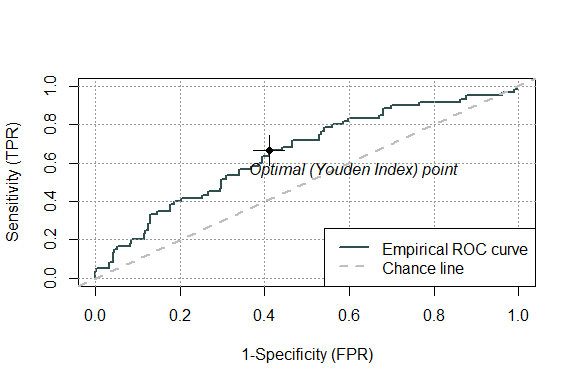# Changing color
plot(roc_binormal, YIndex = F,
values = F, col = c(2,4))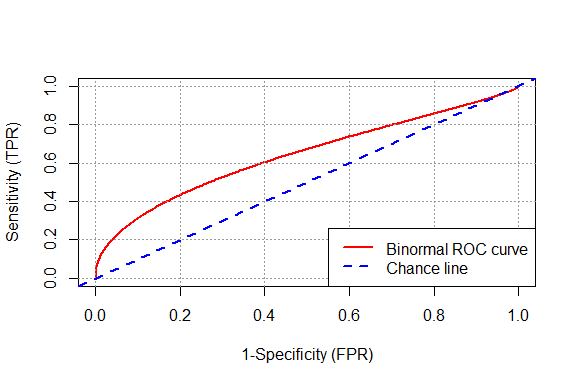# Other options
plot(roc_nonparametric, YIndex = F,
values = F, legend = F)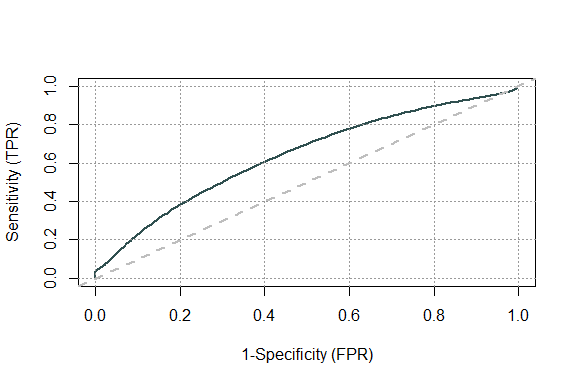Trying a better model:

## first, fit a logistic model
logistic.model <- glm(as.factor(dtest)~
chol+age+bmi,
data = Diabetes,
family = "binomial")

## make the score and class
class <- logistic.model$y # score = log odds score <- logit(logistic.model$fitted.values)

## rocit object
rocit_emp <- rocit(score = score,
class = class,
method = "emp")
rocit_bin <- rocit(score = score,
class = class,
method = "bin")
rocit_non <- rocit(score = score,
class = class,
method = "non")

summary(rocit_emp)
#>
#>  Empirical ROC curve
#>  Number of postive responses :  325
#>  Number of negative responses :  58
#>  Area under curve :  0.783395225464191
summary(rocit_bin)
#>
#>  Binormal ROC curve
#>  Number of postive responses :  325
#>  Number of negative responses :  58
#>  Area under curve :  0.785449952447776
summary(rocit_non)
#>
#>  Non-parametric ROC curve
#>  Number of postive responses :  325
#>  Number of negative responses :  58
#>  Area under curve :  0.773854658684883

## Plot ROC curve
plot(rocit_emp, col = c(1,"gray50"),
legend = FALSE, YIndex = FALSE)
lines(rocit_bin$TPR~rocit_bin$FPR,
col = 2, lwd = 2)
lines(rocit_non$TPR~rocit_non$FPR,
col = 4, lwd = 2)
legend("bottomright", col = c(1,2,4),
c("Empirical ROC", "Binormal ROC",
"Non-parametric ROC"), lwd = 2)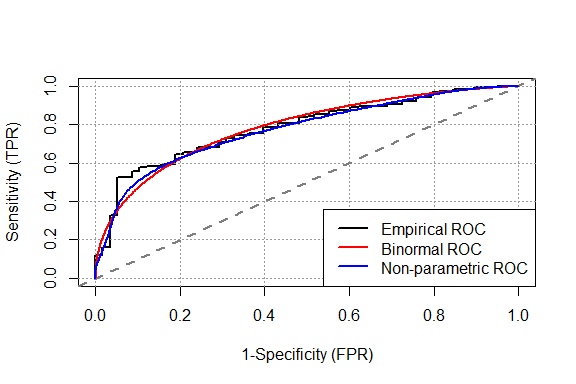Confidence interval of AUC:

# Default
ciAUC(rocit_emp)
#>
#>    estimated AUC : 0.783395225464191
#>    AUC estimation method : empirical
#>
#>    CI of AUC
#>    confidence level = 95%
#>    lower = 0.729587978876528     upper = 0.837202472051854
ciAUC(rocit_emp, level = 0.9)
#>
#>    estimated AUC : 0.783395225464191
#>    AUC estimation method : empirical
#>
#>    CI of AUC
#>    confidence level = 90%
#>    lower = 0.738238760649477     upper = 0.828551690278905

# DeLong method
ciAUC(rocit_bin, delong = TRUE)
#>
#>    estimated AUC : 0.785449952447776
#>    AUC estimation method : binormal
#>
#>    CI of AUC, delong method of variance used
#>    confidence level = 95%
#>    lower = 0.727341865006208     upper = 0.843558039889344

# logit and inverse logit applied
ciAUC(rocit_bin, delong = TRUE,
logit = TRUE)
#>
#>    estimated AUC : 0.785449952447776
#>    AUC estimation method : binormal
#>
#>    CI of AUC, delong method of variance used, logit tranformation applied
#>    confidence level = 95%
#>    lower = 0.72169723187101     upper = 0.837879081307966

# bootstrap method
set.seed(200)
ciAUC_boot <- ciAUC(rocit_non,
level = 0.9, nboot = 200)
print(ciAUC_boot)
#>
#>    estimated AUC : 0.773854658684883
#>    AUC estimation method : non-parametric
#>
#>    bootstrap CI of AUC with 200 boot samples
#>    confidence level = 90%
#>    lower = 0.738588859416446     upper = 0.826931034482759

Confidence interval of ROC curve:

data("Loan")
score <- Loan$Score class <- ifelse(Loan$Status == "CO", 1, 0)
rocit_emp <- rocit(score = score,
class = class,
method = "emp")
rocit_bin <- rocit(score = score,
class = class,
method = "bin")
# --------------------------
ciROC_emp90 <- ciROC(rocit_emp,
level = 0.9)
set.seed(200)
ciROC_bin90 <- ciROC(rocit_bin,
level = 0.9, nboot = 200)
plot(ciROC_emp90, col = 1,
legend = FALSE)
lines(ciROC_bin90$TPR~ciROC_bin90$FPR,
col = 2, lwd = 2)
lines(ciROC_bin90$LowerTPR~ciROC_bin90$FPR,
col = 2, lty = 2)
lines(ciROC_bin90$UpperTPR~ciROC_bin90$FPR,
col = 2, lty = 2)
legend("bottomright", c("Empirical ROC",
"Binormal ROC",
"90% CI (Empirical)",
"90% CI (Binormal)"),
lty = c(1,1,2,2), col =
c(1,2,1,2), lwd = c(2,2,1,1))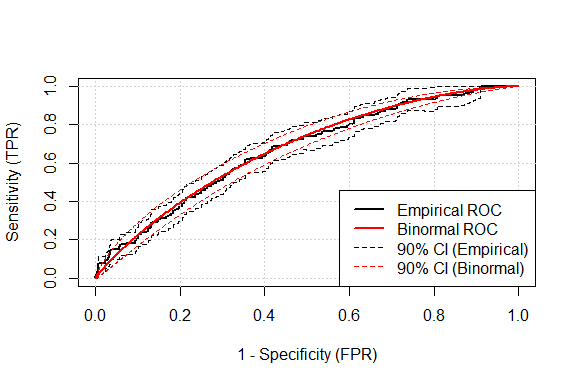Empirical ROC curve with 90% CI

Options available for plotting ROC curve with CI

class(ciROC_emp90)
#>  "rocci"

KS plot: KS plot shows the cumulative density functions $$F(c)$$ and $$G(c)$$ in the positive and negative populations. If the positive population have higher value, then negative curve ($$F(c)$$) ramps up quickly. The KS statistic is the maximum difference of $$F(c)$$ and $$G(c)$$.

data("Diabetes")
logistic.model <- glm(as.factor(dtest)~
chol+age+bmi,
data = Diabetes,
family = "binomial")
class <- logistic.model$y score <- logistic.model$fitted.values
# ------------
rocit <- rocit(score = score,
class = class) #default: empirical
kplot <- ksplot(rocit)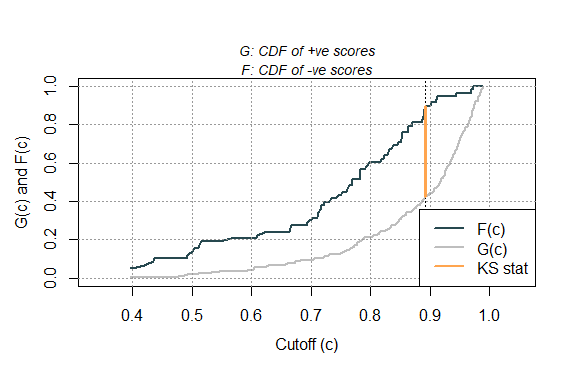KS plot

message("KS Stat (empirical) : ",
kplot$KS stat) #> KS Stat (empirical) : 0.471936339522546 message("KS Stat (empirical) cutoff : ", kplot$KS Cutoff)
#> KS Stat (empirical) cutoff : 0.892084996383686

## Gains table

Gains table is a useful tool used in direct marketing. The observations are first rank ordered and certain number of buckets are created with the observations. The gains table shows several statistics associated with the buckets. This package includes gainstable function that creates gains table containing ngroup number of groups or buckets. The algorithm first orders the score variable with respect to score variable. In case of tie, it class becomes the ordering variable, keeping the positive responses first. The algorithm calculates the ending index in each bucket as $$round((length(score) / ngroup) * (1:ngroup))$$. Each bucket should have at least 5 observations.

If buckets’ end index are to be ended at desired level of population, then breaks should be specified. If specified, it overrides ngroup and ngroup is ignored. breaks by default always includes 100. If whole number does not exist at specified population, nearest integers are considered. Following stats are computed:

• Obs: Number of observation in the group.
• CObs: Cumulative number of observations up to the group.
• Depth: Cumulative population depth up to the group.
• Resp: Number of (positive) responses in the group.
• CResp: Cumulative number of (positive) responses up to the group.
• RespRate: (Positive) response rate in the group.
• CRespRate: Cumulative (positive) response rate up to the group
• CCapRate: Cumulative overall capture rate of (positive) responses up to the group.
• Lift: Lift index in the group. Calculated as GroupResponseRate / OverallResponseRate.
• CLift: Cumulative lift index up to the group.
data("Loan")
class <- Loan$Status score <- Loan$Score
# ----------------------------
gtable15 <- gainstable(score = score,
class = class,
negref = "FP",
ngroup = 15)

rocit object can be passed

rocit_emp <- rocit(score = score,
class = class,
negref = "FP")
gtable_custom <- gainstable(rocit_emp,
breaks = seq(1,100,15))
# ------------------------------
print(gtable15)
#>    Bucket Obs CObs Depth Resp CResp RespRate CRespRate CCapRate  Lift
#> 1       1  60   60 0.067   20    20    0.333     0.333    0.153 2.290
#> 2       2  60  120 0.133   11    31    0.183     0.258    0.237 1.260
#> 3       3  60  180 0.200   12    43    0.200     0.239    0.328 1.374
#> 4       4  60  240 0.267   14    57    0.233     0.238    0.435 1.603
#> 5       5  60  300 0.333   11    68    0.183     0.227    0.519 1.260
#> 6       6  60  360 0.400   13    81    0.217     0.225    0.618 1.489
#> 7       7  60  420 0.467    9    90    0.150     0.214    0.687 1.031
#> 8       8  60  480 0.533    7    97    0.117     0.202    0.740 0.802
#> 9       9  60  540 0.600    5   102    0.083     0.189    0.779 0.573
#> 10     10  60  600 0.667    9   111    0.150     0.185    0.847 1.031
#> 11     11  60  660 0.733    4   115    0.067     0.174    0.878 0.458
#> 12     12  60  720 0.800    7   122    0.117     0.169    0.931 0.802
#> 13     13  60  780 0.867    3   125    0.050     0.160    0.954 0.344
#> 14     14  60  840 0.933    6   131    0.100     0.156    1.000 0.687
#> 15     15  60  900 1.000    0   131    0.000     0.146    1.000 0.000
#>    CLift
#> 1  2.290
#> 2  1.775
#> 3  1.641
#> 4  1.632
#> 5  1.557
#> 6  1.546
#> 7  1.472
#> 8  1.388
#> 9  1.298
#> 10 1.271
#> 11 1.197
#> 12 1.164
#> 13 1.101
#> 14 1.071
#> 15 1.000
print(gtable_custom)
#>   Bucket Obs CObs Depth Resp CResp RespRate CRespRate CCapRate  Lift CLift
#> 1      1   9    9  0.01    5     5    0.556     0.556    0.038 3.817 3.817
#> 2      2 135  144  0.16   33    38    0.244     0.264    0.290 1.679 1.813
#> 3      3 135  279  0.31   26    64    0.193     0.229    0.489 1.323 1.576
#> 4      4 135  414  0.46   26    90    0.193     0.217    0.687 1.323 1.494
#> 5      5 135  549  0.61   13   103    0.096     0.188    0.786 0.662 1.289
#> 6      6 135  684  0.76   18   121    0.133     0.177    0.924 0.916 1.215
#> 7      7 135  819  0.91    7   128    0.052     0.156    0.977 0.356 1.074
#> 8      8  81  900  1.00    3   131    0.037     0.146    1.000 0.254 1.000
plot(gtable15, type = 1)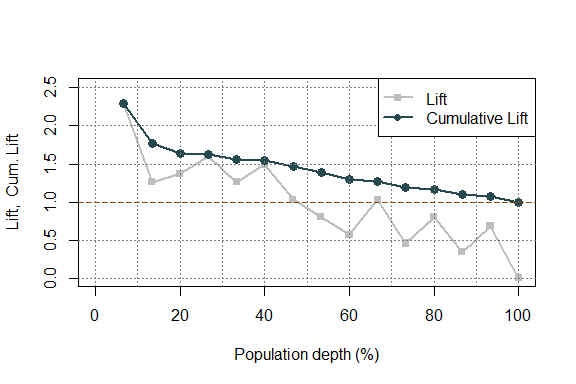Lift and Cum. Lift plot